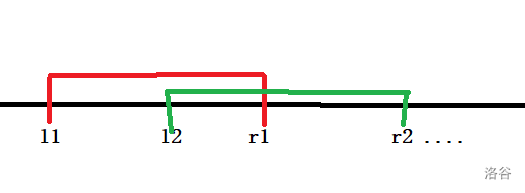### 思路

（题目中%1e9+7 不就表明了这一点）

（“因此” 好难想）

### 性质：

• 如果设 $(l1,r1)$,$(l2,r2)$分别为两条连边且 $l1<r1$,$l2<r2$

• 我们来分析连通块$l2,r2$为连通块 B 最左侧和右侧的点

### 从性质出发的做法

$g_i$表示 $i$个点的连线总方案